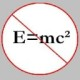You are currently browsing the tag archive for the ‘euclidean’ tag.

High-school students and undergraduates are (almost) always taught the following definition of an equivalence relation.

A binary relation$R$ on a set$A$ is an equivalence iff it satisfies

• the reflexive property: for all$a$  in$A$,$a R a$,
• the symmetric property: for all$a, b$ in$A$, if$a R b$, then$b R a$, and
• the transitive property: for all$a, b, c$ in$A$, if$a R b$ and$b R c$, then$a R c$.

However, there is another formulation of an equivalence relation that one usually doesn’t hear about, as far as I know. And, it is the following one.

A binary relation$R$ on a set$A$ is an equivalence iff it satisfies

• the reflexive property: for all$a$  in$A$,$a R a$, and
• the euclidean property: for all$a, b, c$ in$A$, if$a R b$ and$a R c$, then$b R c$.

Exercise:  Show that a binary relation$R$ on a set$A$ is reflexive, symmetric and transitive iff it is reflexive and euclidean.

Blog Stats

• 338,531 hitsprof drd horia orasa… on My first postprof dr mircea orasa… on Inequality with lognotedscholar on Self-referential Paradoxes, In…prof dr mircea orasa… on Inequality with logprof dr mircea orasa… on Inequality with logprof dr mircea orasa… on 2010 in reviewkenji on Basic category theory, Iprof dr mircea orasa… on Solution to POW-10: Another ha…prof drd horia orasa… on Continued fraction for eprof drd horia orasa… on Inequality with logprof dr mircea orasa… on Solution to POW-12: A graph co…prof dr mircea orasa… on The Character of Physical…prof dr mircea orasa… on Milk and Tea puzzleprof drd horia orasa… on Inequality with logprof dr mircea orasa… on The Character of Physical…